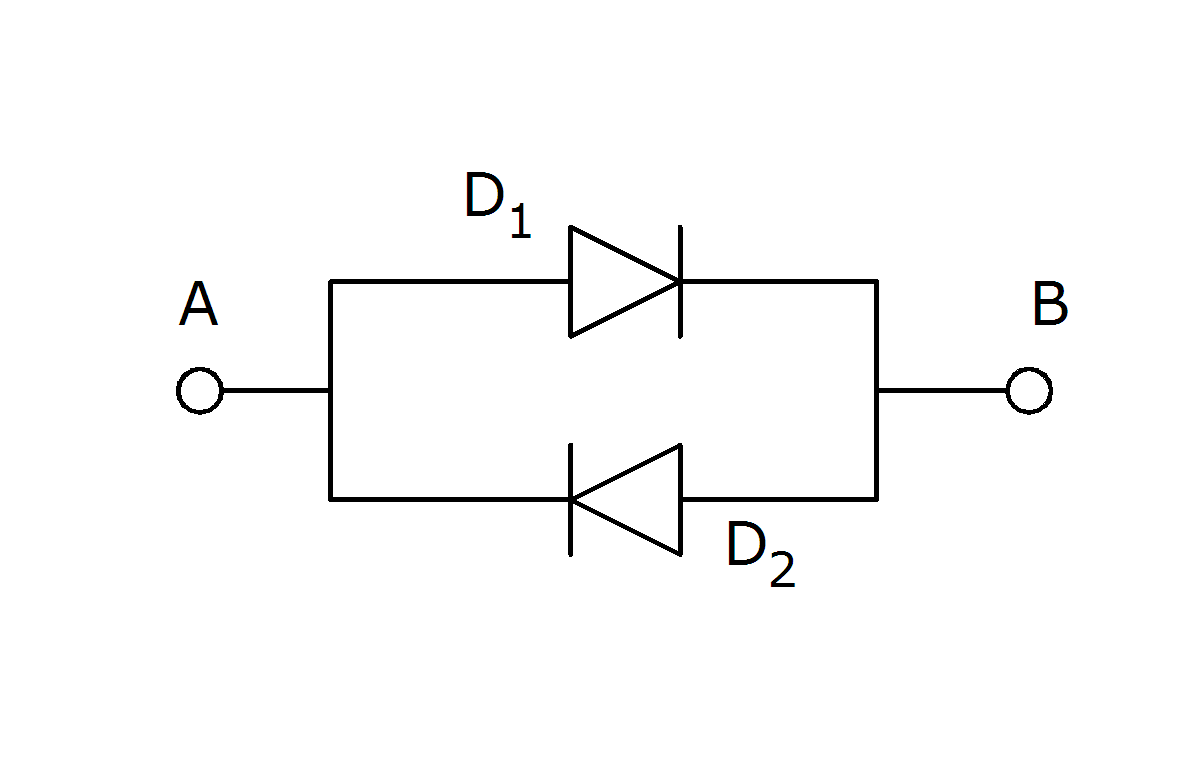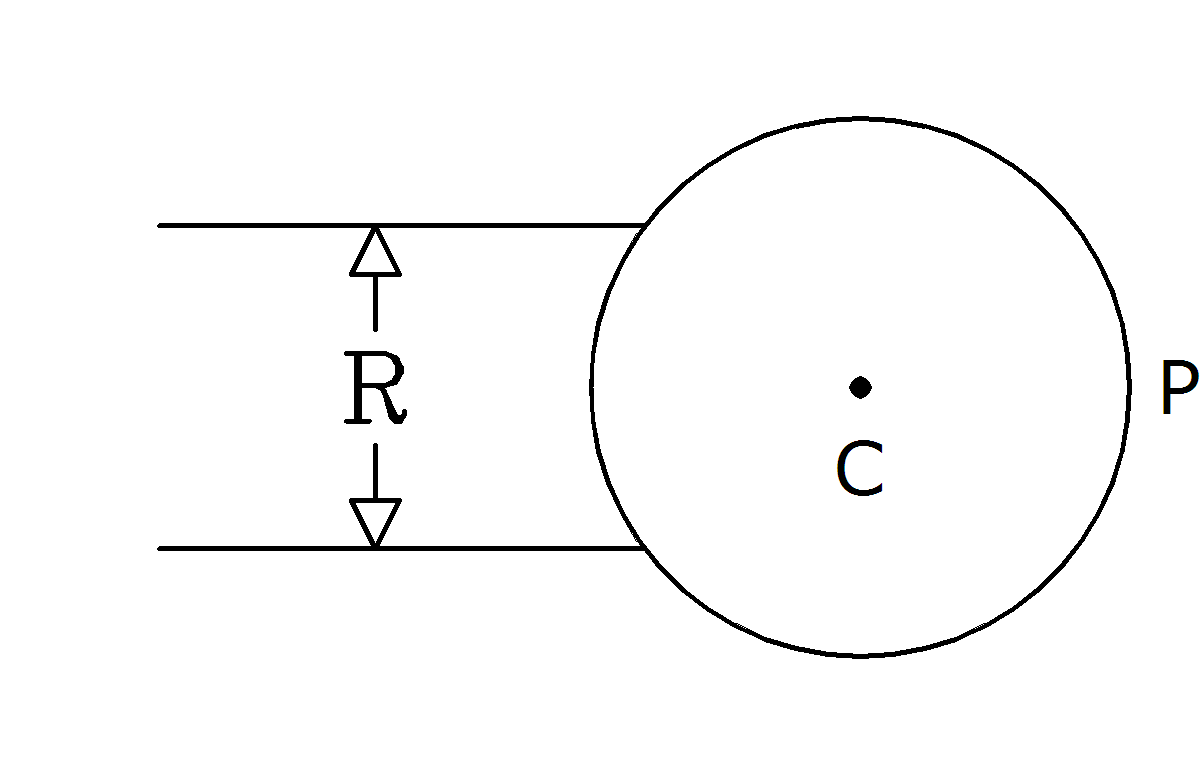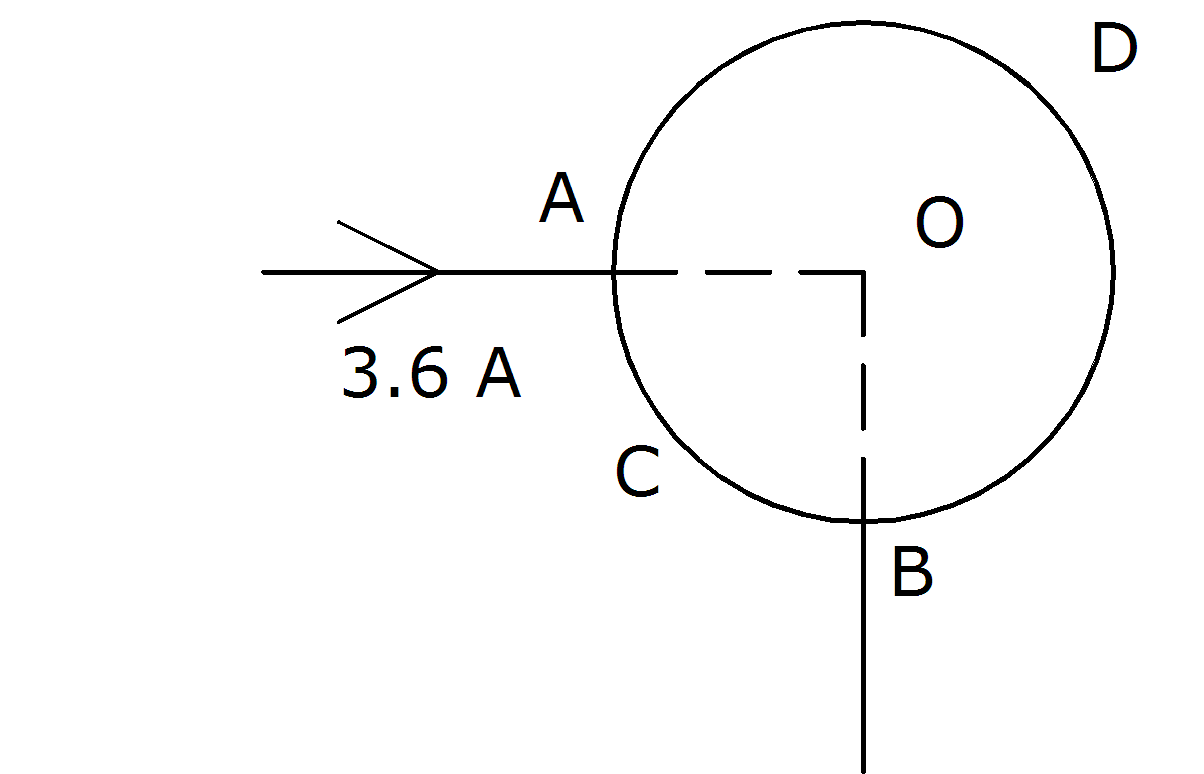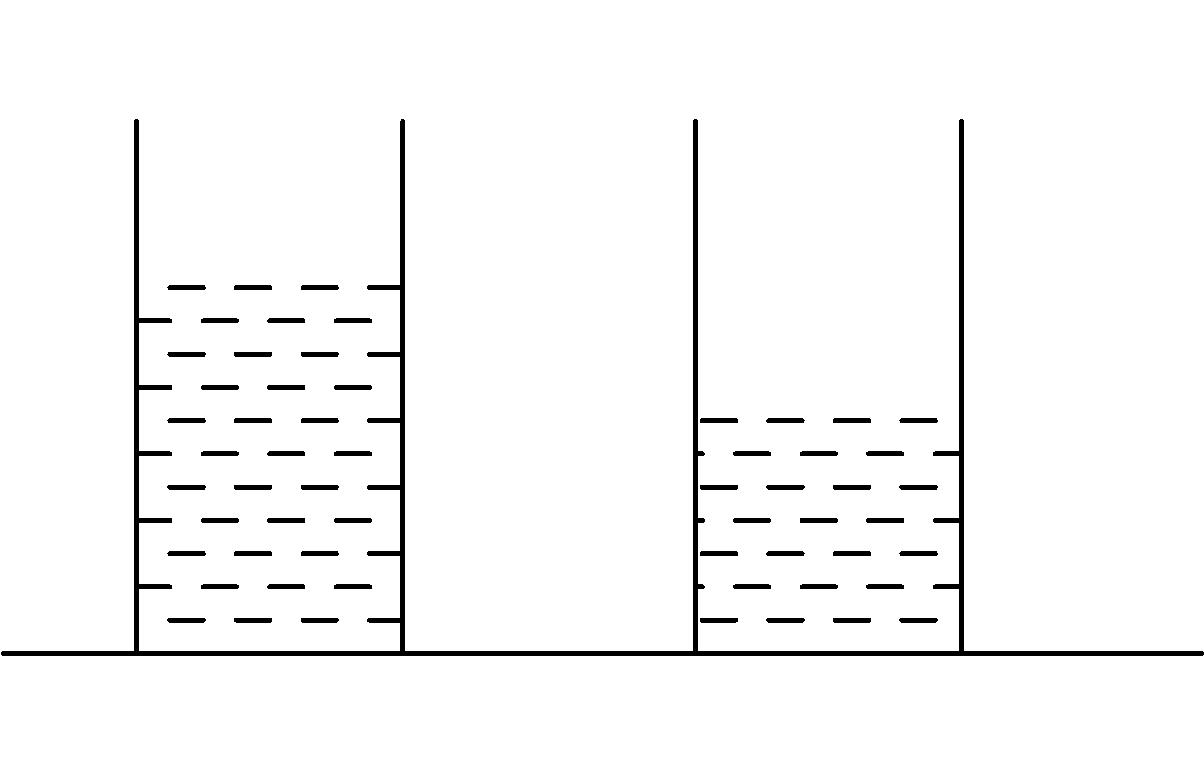PHYSICS

 Time: ONE Hour Max. Marks: 60

 Answers must be written either in English or the medium of instruction of the candidate in high school. Answer all the questions in the booklets provided for the purpose. There will be no negative marking. The relevant working or the argument in arriving at an answer has to be included in your answer. Use of calculators or graph papers is not permitted. Questions in Part A carry 6 marks each and questions in part B carry 3 marks each.

Part A

1.Two liquids of masses 600 g and 500 g and densities 0.6 gcm-3 and 1.5 gcm-3 are combined to form a homogenous mixture. A solid block of mass 420 g just floats in this mixture. Find the density of the block material. If the equal volumes of the two liquids were mixed instead, would this block float in that mixture? If it floats find the fraction of the block that is visible above the mixture.

2.A lens of focal length f produces an image of an object located 30 cm on one side of it at a distance of 60 cm on the other side. If the lens is replaced by another of 3f/4, where would the image form? If the image has to form at the earlier position by what distance should the object be shifted?

3.The devices D1 and D2 shown (below left) are called diodes and have an interesting property. When current flows in the direction of the arrow the resistance is low and is called forward bias resistance. If the current were to flow in the opposite direction the resistance is high and it is called reverse bias resistance. For D1 forward bias resistance is 20 Ohm and reverse bias resistance is 600 Ohm. For D2 forward bias resistance is 30 Ohm and reverse bias resistance is 200 Ohm. Find the equivalent resistance between A and B if (i) A is at a higher potential (ii) B is at a higher potential

4.Rays of light are incident symmetrically on a sphere of radius R as shown (below middle). Determine the refractive index of the sphere material if they meet at P (You need to write the correct expression for refractive index). Can the rays be made to meet at C, the center of the sphere. If not, how should the rays be incident to meet at C.5.If the measured potential difference between A and B is 5.4 V, Find the rate of heat production in the ring (above right). Also find the rate of heat production in sections ACB and ADB.

 6.Two identical rectangular tanks are connected by a small tube at the bottom. In the left tank contains liquid of density d1=800 kg/m3 to a height h1=30 cm. The right tank contains liquid of density d2=1600 kg/m3, to a height h2=20 cm. The two liquids don't mix. a) Find the heights of the respective columns, if a small tube connects them at the bottom. b) Where should a horizontal tube be connected, without causing any flow between the tanks?Part B

7.Two candles of identical lengths and different thicknesses were lit. Half of the thin candle and one third of the thick candle could be seen to burn down in 15 minutes. How long after the candles had been lit was the ratio of their lengths 4.

8.The average daily loss of water of a human body is 0.8 kg, evaporated by the body. How much food has to be consumed in order to compensate for the loss of energy due to the evaporation if human body can use 70% of the energy provided by the decomposition of food. The heat of combustion of food is 6,000 kJ/kg, the heat of vaporisation of water is 2440 kJ/kg

9.A train of length 300 m passes through two tunnels with a gap of 400 m between them .The tunnels are of length 800 m and 600 m respectively. From the moment it just emerges from the first tunnel to the moment it just disappears in to the second tunnel time elapsed is 60 s. Find the time elapsed between the moments the train just enters the first tunnel to the moment it just leaves the second tunnel.

10.Fiber optic cable of length L has an inner core surrounded by an outer jacket. Light can travel along the length of the cable through the inner and outer portions. The refractive indices of the material of the inner and outer portions are m 1 and m 2. Find the time interval between the moments when a light pulse entering one end of the cable arrives at the other end traveling through the inner and outer portions.

11.A person's heart does approximately a work of 1.6 J during a beat. How much work does it do during a day? By how much does the temperature of 10 kg of water rise if it absorbs this much of energy?

12.Find the ratio Ei/Ef, where Ei and Ef are the energies of a neutron before and after interaction with the nuclei of the moderator in a nuclear reactor.

13.A stone projected vertically upward on some planet reaches a maximum height h. Find the ratio of times after projection when it is at a height of 5h/9.

14.A horizontal conductor is oriented north south and carries some current. A positively charged particle located vertically above it and having a velocity directed northward experiences an upward force. What is the direction of the force if this charged particle were located to the east of the conductor and had a velocity directed towards the conductor.